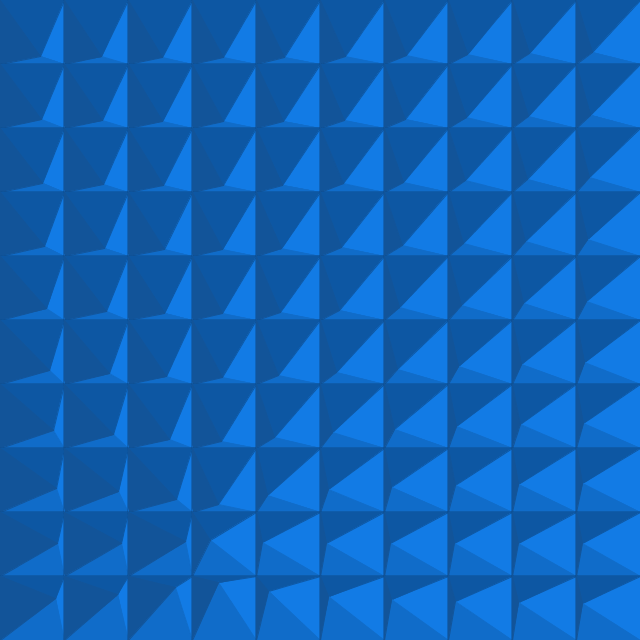# Art › Moving Pyramids## Project Code

This code is written and rendered using Processing (v3), a quick and easy graphical sketchbook engine written in Java
``````class GridItem {
PVector location;
PVector velocity;
PVector acceleration;

float x;
float y;
float box_size = 64.0;

GridItem(float x_, float y_) {
x = x_;
y = y_;

location = new PVector(
random(2, (box_size - 2)),
random(2, (box_size - 2))
);
velocity = new PVector(0, 0);
acceleration = PVector.random2D();
acceleration.mult(0.3);
}

void update() {
//updateBasic();
updateMouseFollow();
}

void updateBasic() {
// movement
velocity.limit(1);

float scale_x = norm(location.x, 0, box_size);
float scale_y = norm(location.y, 0, box_size);

if((scale_x <= 0) || (scale_x >= 1)) {
velocity.x *= -1;
acceleration.x *= -1;
}

if((scale_y <= 0) || (scale_y >= 1)) {
velocity.y *= -1;
acceleration.y *= -1;
}
}

void updateMouseFollow() {
float center_worldX = ((box_size * x) + (box_size * 0.5));
float center_worldY = ((box_size * y) + (box_size * 0.5));

float max_radius = (box_size * 0.4);
float center_distance = dist(
center_worldX,
center_worldY,
followX,
followY
);

float angle = atan2((followY - center_worldY), (followX - center_worldX));

location.x = ((box_size * 0.5) + (cos(angle) * real_radius));
location.y = ((box_size * 0.5) + (sin(angle) * real_radius));
}

void draw() {
color light = color(18,123,229);
color lightish = color(16, 108, 201);
color dark = color(13, 87, 163);
color darker = color(18, 84, 153);
color border = color(11, 76, 142);

pushMatrix();

translate(
(x * 64),
(y * 64)
);

pushStyle();
fill(border);
strokeWeight(2);
stroke(border);
rect(0, 0, 64, 64);
popStyle();

int borderSize = 1;

// right
pushStyle();
beginShape();

if(borderSize > 0) {
stroke(light);
strokeWeight(borderSize);
} else {
noStroke();
}

fill(light);

vertex(box_size, 0);
vertex(box_size, box_size);
vertex(location.x, location.y);

endShape(CLOSE);
popStyle();

// top
pushStyle();
beginShape();

if(borderSize > 0) {
stroke(dark);
strokeWeight(borderSize);
} else {
noStroke();
}

fill(dark);

vertex(0, 0);
vertex(box_size, 0);
vertex(location.x, location.y);

endShape(CLOSE);
popStyle();

// bottom
pushStyle();
beginShape();

if(borderSize > 0) {
stroke(lightish);
strokeWeight(borderSize);
} else {
noStroke();
}

fill(lightish);

vertex(0, box_size);
vertex(box_size, box_size);
vertex(location.x, location.y);

endShape(CLOSE);
popStyle();

// left
pushStyle();
beginShape();

if(borderSize > 0) {
stroke(darker);
strokeWeight(borderSize);
} else {
noStroke();
}

fill(darker);

vertex(0, 0);
vertex(0, box_size);
vertex(location.x, location.y);

endShape(CLOSE);
popStyle();

popMatrix();
}
}
``````
``````GridItem[][] grid_items;

int loopFollowAfterFrames = (40 * 4);
float followX, followY;
float followBorder = 100;

void setup() {
size(640, 640);

grid_items = new GridItem;

for(int y = 0; y < 10; y++) {
for(int x = 0; x < 10; x++) {
grid_items[ y ][ x ] = new GridItem(x, y);
}
}
}

void draw() {
background(color(255, 255, 255));

updateFollowPosition();

for(int y = 0; y < 10; y++) {
for(int x = 0; x < 10; x++) {
grid_items[ y ][ x ].update();
grid_items[ y ][ x ].draw();
}
}

if(frameCount == 50) { save("preview.png"); }
}

void updateFollowPosition() {
float frameProgress = float((frameCount % loopFollowAfterFrames));
float quarterProgress = (float(loopFollowAfterFrames) * 0.25);
float realProgress = (frameProgress / quarterProgress);

float widthWithoutFollowBorders = (float(width) - (followBorder * 2));
float heightWithoutFollowBorders = (float(height) - (followBorder * 2));

if(realProgress < 1) {
followX = followBorder;
followY = (followBorder + (heightWithoutFollowBorders * realProgress));
} else if(realProgress < 2) {
followX = (followBorder + (widthWithoutFollowBorders * (realProgress - 1)));
followY = (followBorder + heightWithoutFollowBorders);
} else if(realProgress < 3) {
followX = (followBorder + widthWithoutFollowBorders);
followY = (followBorder + (heightWithoutFollowBorders * (1 - (realProgress - 2))));
} else if(realProgress <= 4) {
followX = (followBorder + (widthWithoutFollowBorders * (1 - (realProgress - 3))));
followY = followBorder;
}
}
``````